•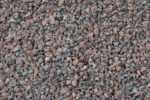•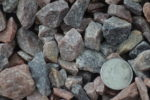•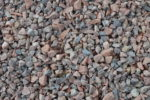# Pink Quartz ¾”

Pink quartz is a great accent to the base of a tree, foundation for a garden, or a trail in your backyard. With this dull pink hue that shines in the light, your clients or neighbors will run to you demanding to know where you obtained such magnificent rock

• Dull pink hue
• Decorative landscape rock
• Our smallest pink quartz
• Brightens flower beds
• Makes a good band name
If you need help finding out your total cubic yardage use one of our calculators below!
Aerial Map Calculator
Area Totals
Current Area
0 ft2
Total Area
0 ft2
Total Measurement: 0 cubic yards
Total Price: \$0
Square Footage Calculator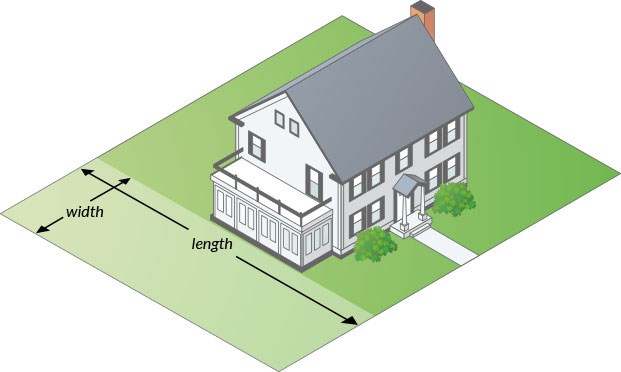#### How to measure your area.

• Area 1
• Area 1
• Area 1
Total Measurement: 0 cubic yards
Total Price: \$0

### How To Measure

#### Square or Rectangle

Measure the Length & Width and multiply.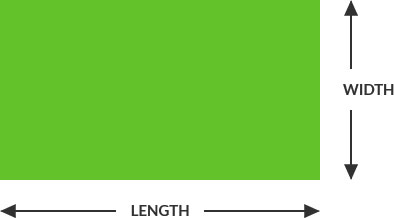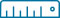Length x Width = Area
(12’ x 24’ = 288 ft2)

#### Right Triangle

Multiply the two sides that contact the right angle and divide by two.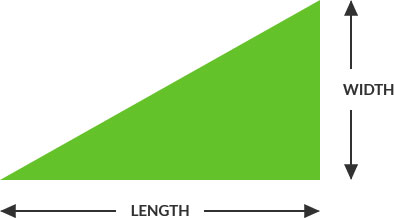Length x Width / 2 = Area
(12’ x 24’ = 288 ÷ 2 = 144 ft2)

#### Circular

Multiply the radius with the radius then multiply by 3.14. Radius is a straight line from the center of a circle to the outside of the circle.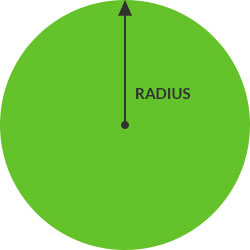##### Full Circle:(6’ x 6’ = 36 x 3.14 = 113 ft2)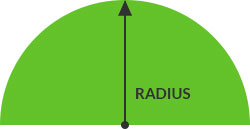##### Half Circle:Divide full circle radius by 2
(6’ x 6’ = 36 x 3.14 = 113 / 2 = 56.5 ft2)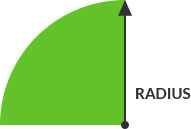##### Quarter Circle:Divide full circle radius by 4
(6’ x 6’ = 36 x 3.14 = 113 / 4 = 28.25 ft2)